# R S Aggarwal Solutions for Class 11 Maths Chapter 7 Linear Inequations in Two Variables

R S Aggarwal Solutions Class 11 Chapter 7 consists of questions based on solving linear inequalities in two variables. The statements of inequalities of the form ax + by + c > 0, ax + by + c < 0, ax + by + c ≥ 0 and ax + by + c ≤ 0 are called linear inequations in two variables x and y. The set of all ordered pairs (x, y) which satisfy the given inequation is the solution set of the inequation. All R S Aggarwal Solutions for Class 11 are explained using step by step problem solving approach. This chapter will help students in understanding the important steps involved in finding the solutions of linear inequations in two variables with the help of graphs.

## Download PDF of R S Aggarwal Solutions for Class 11 Maths Chapter 7 Linear Inequations in Two Variables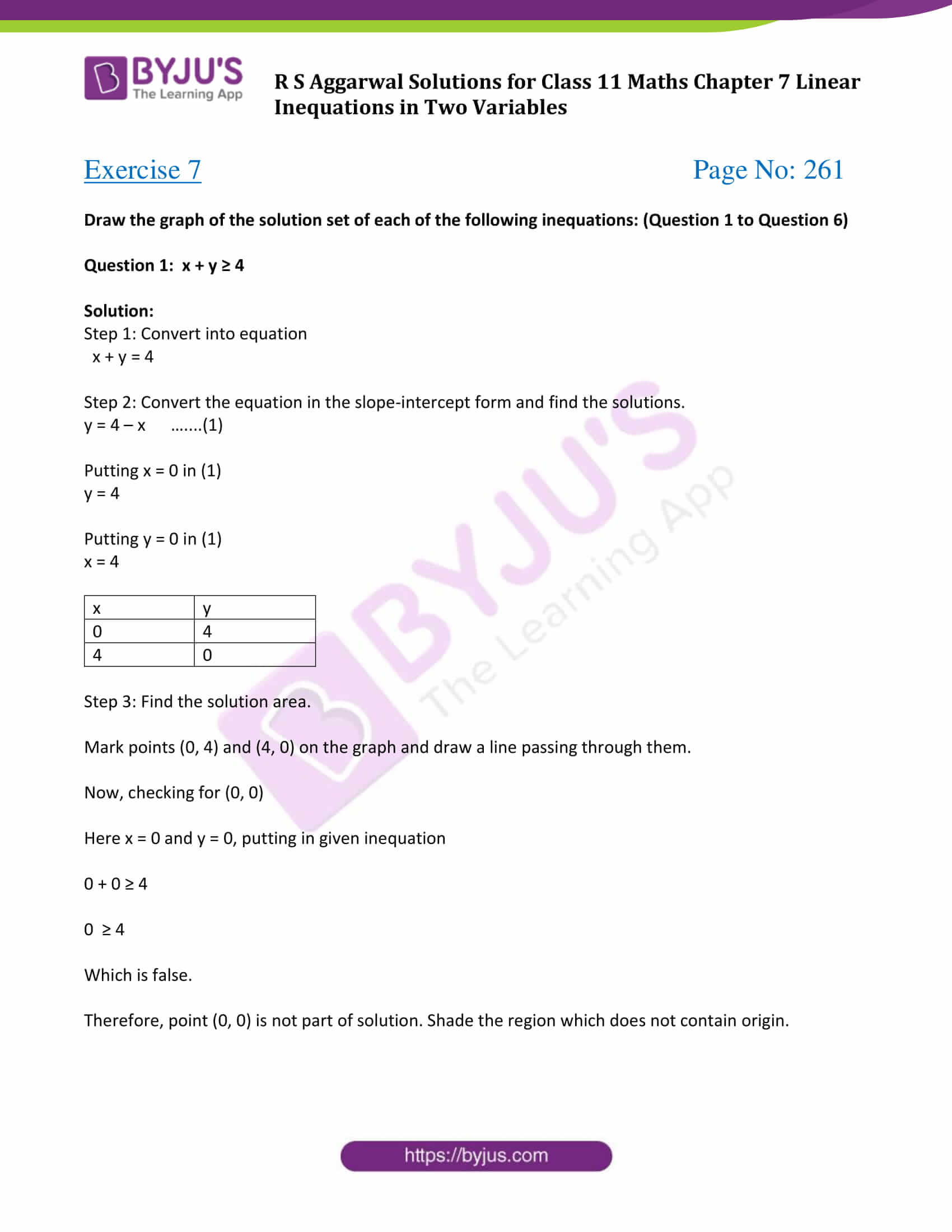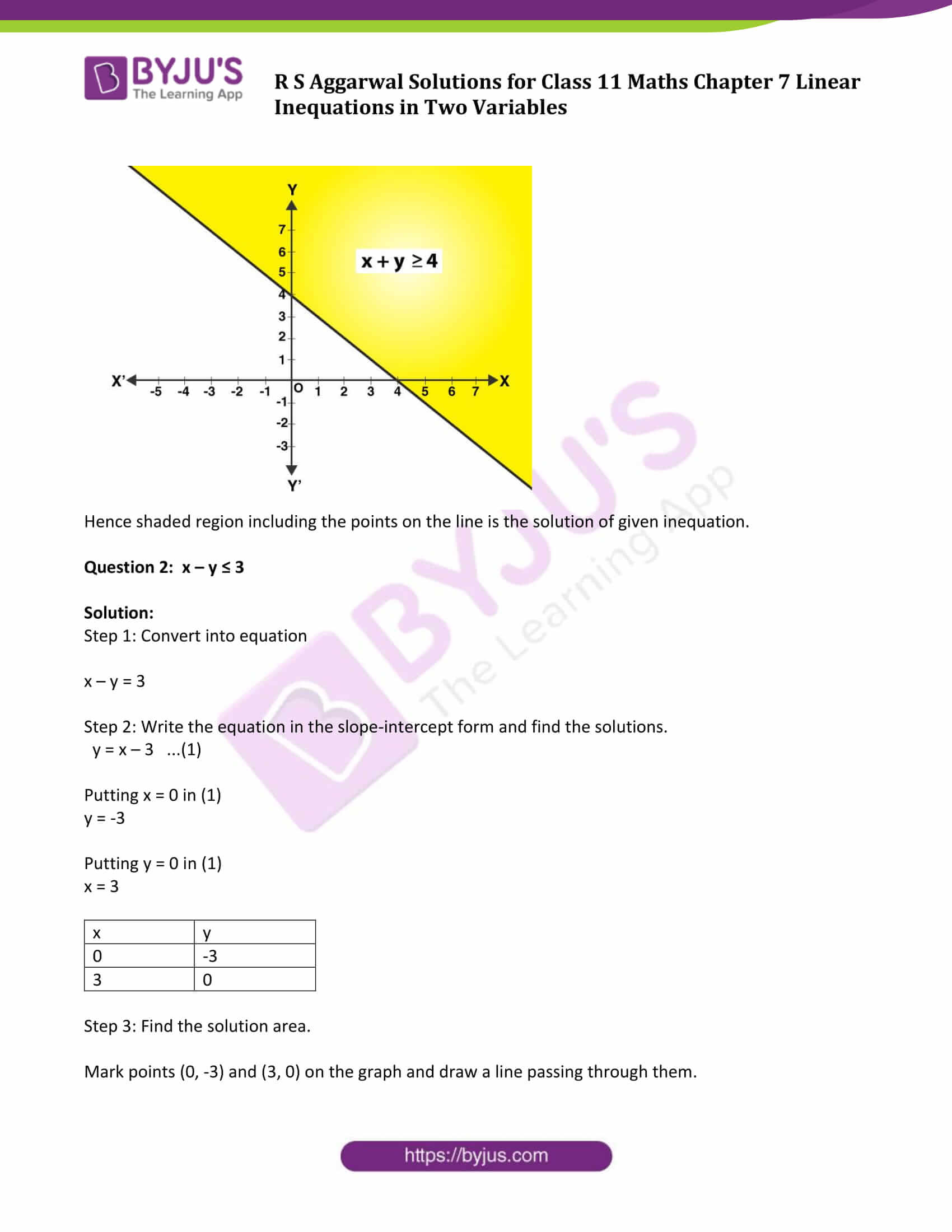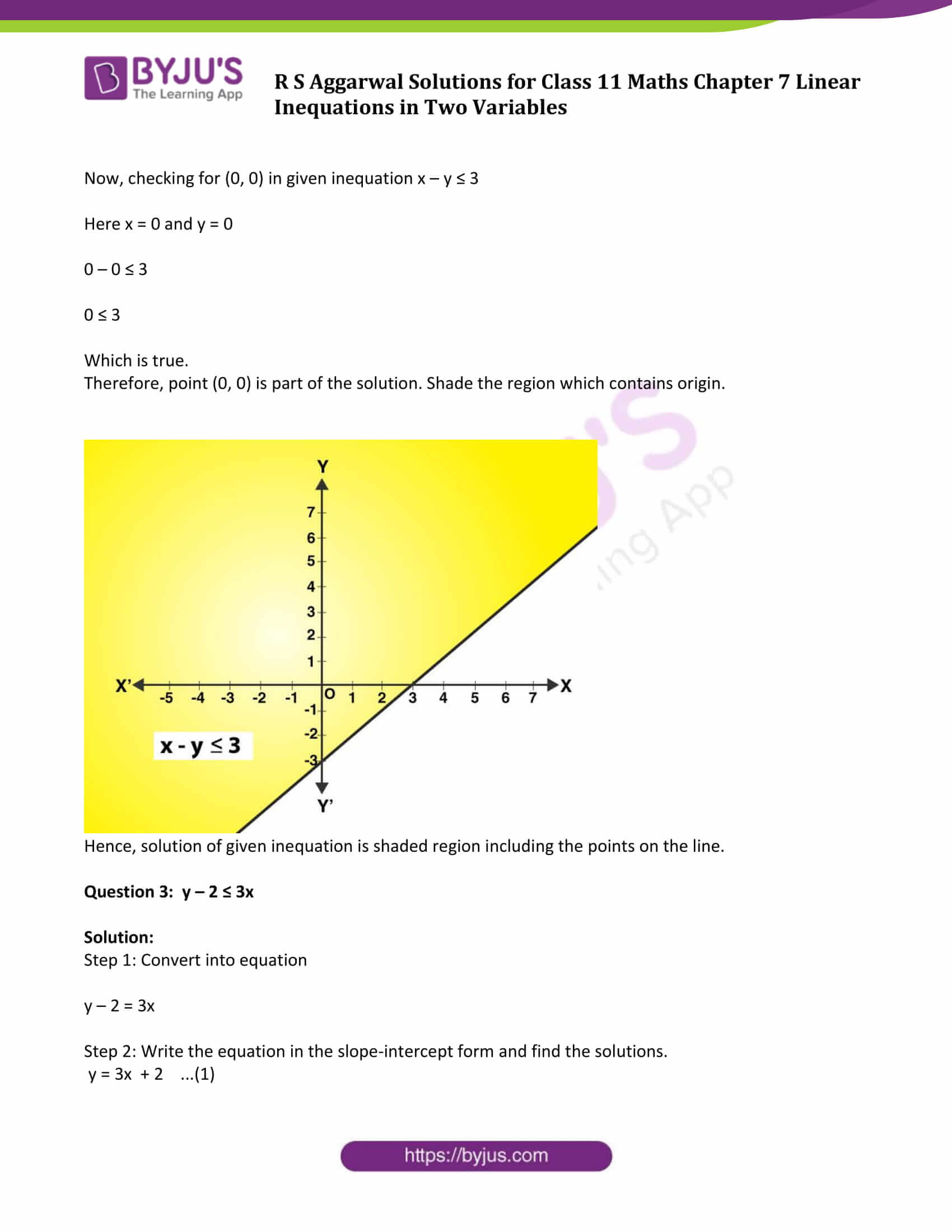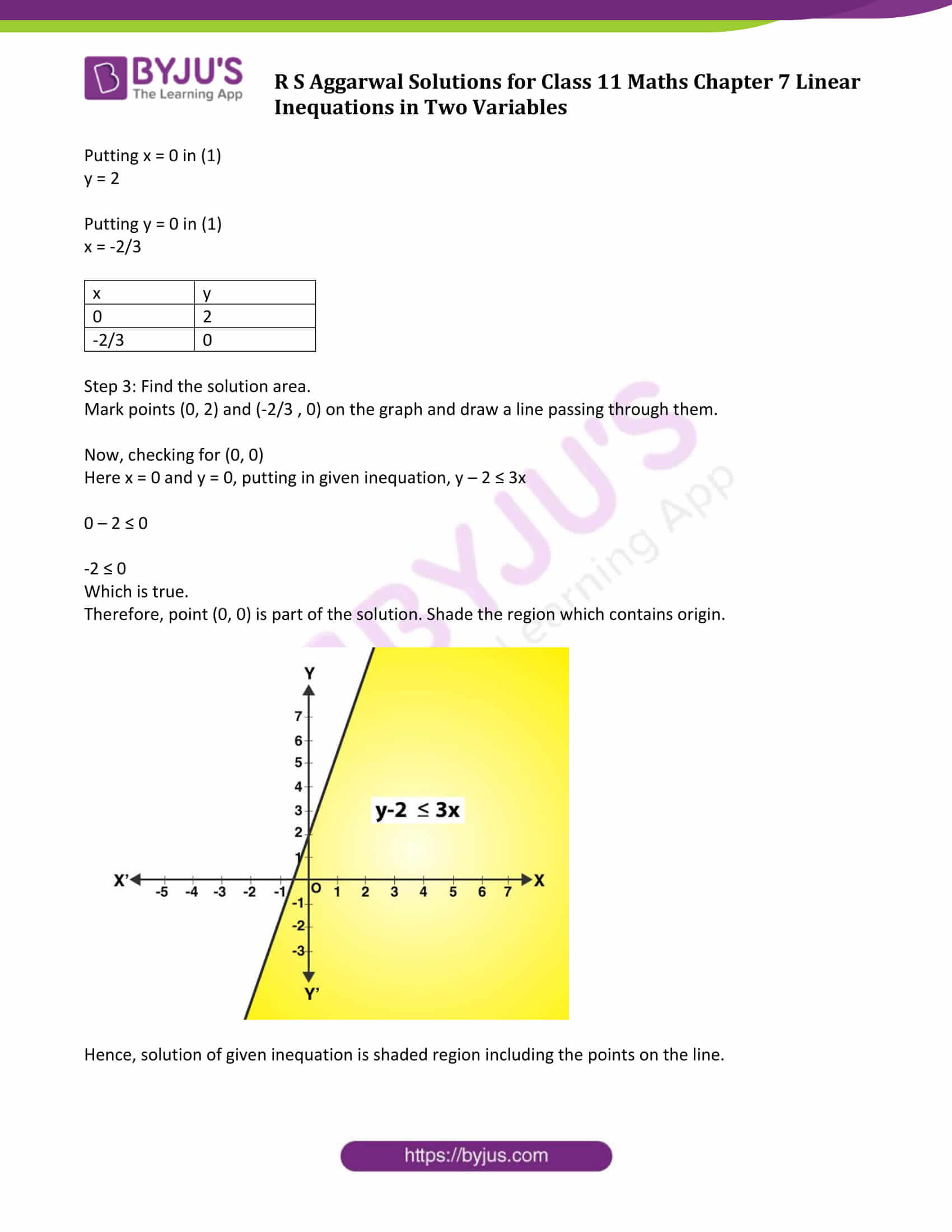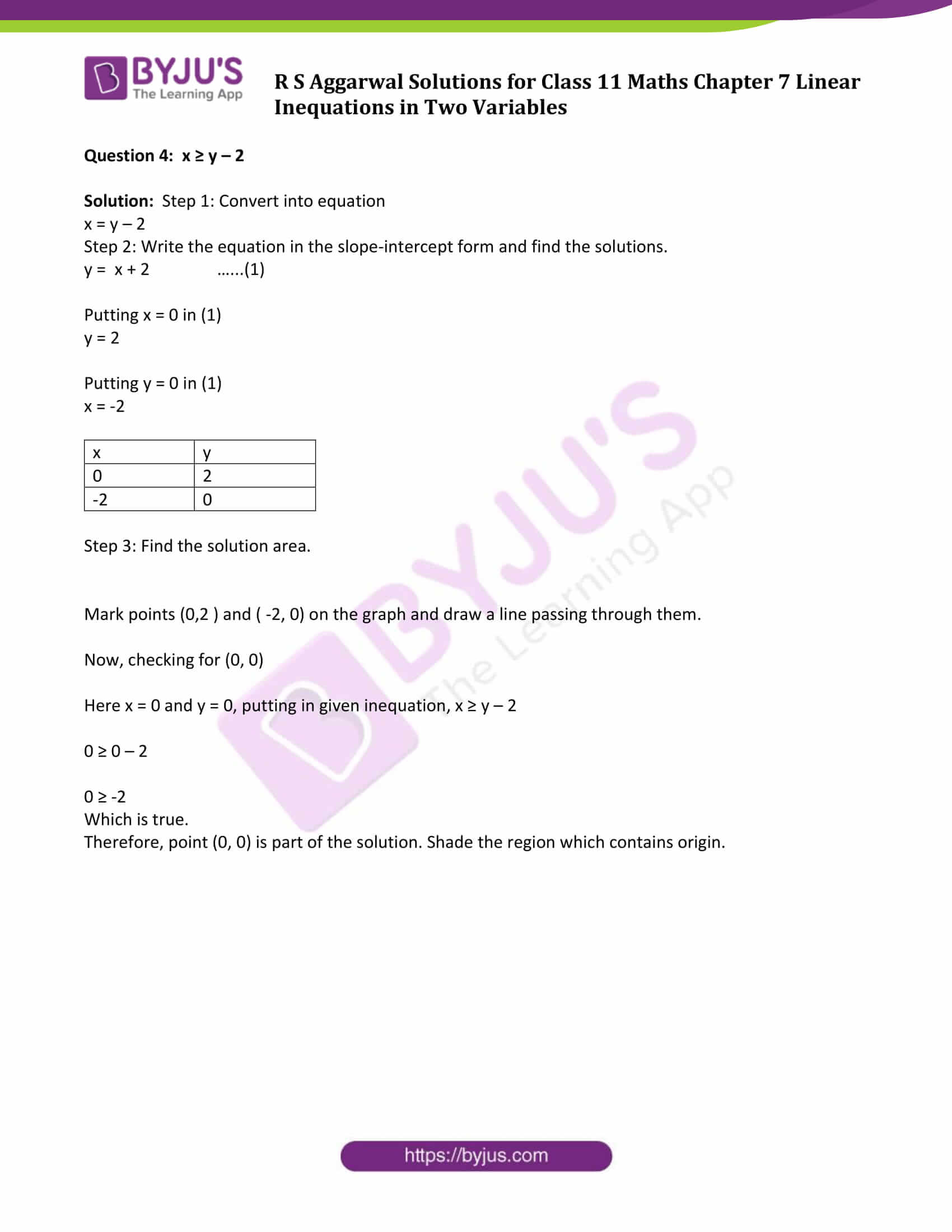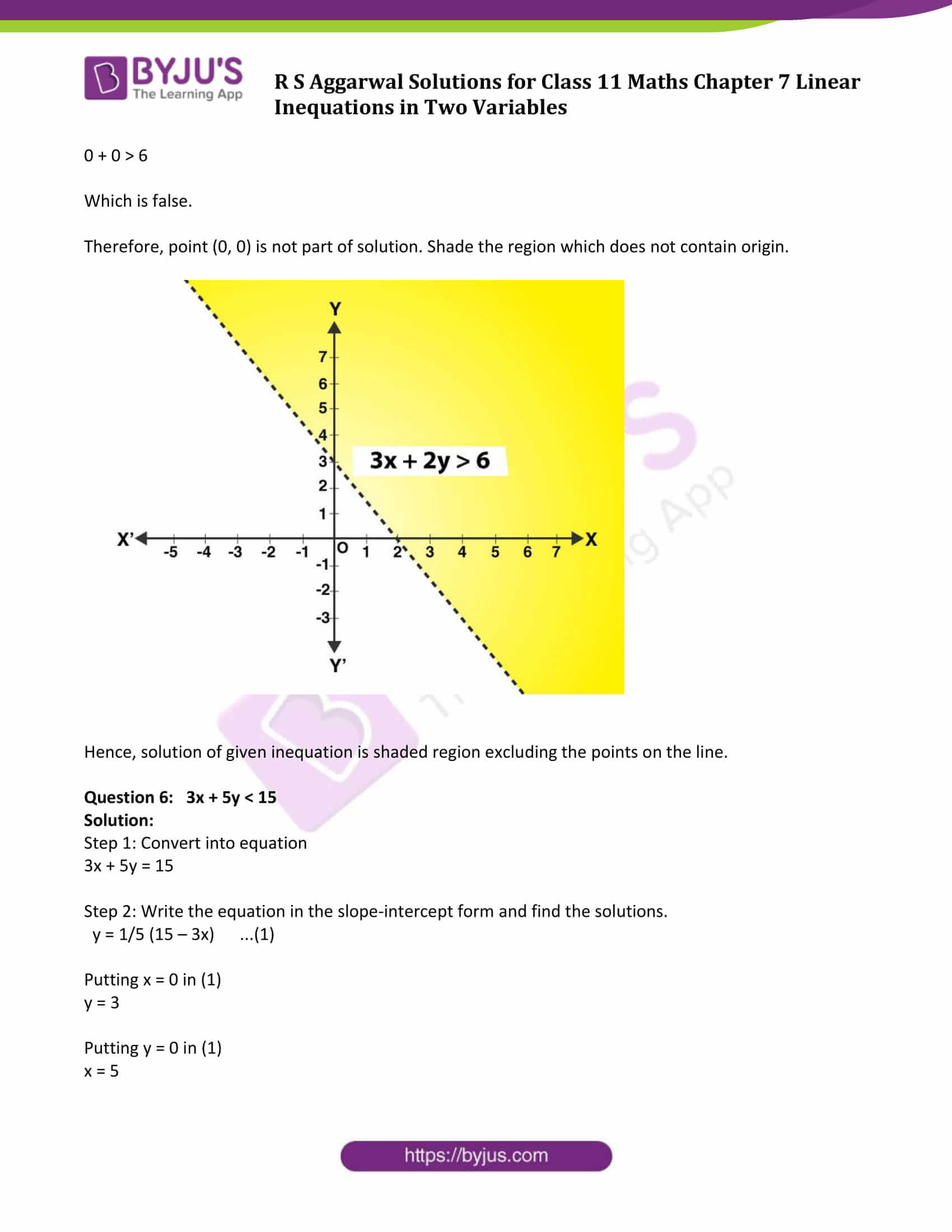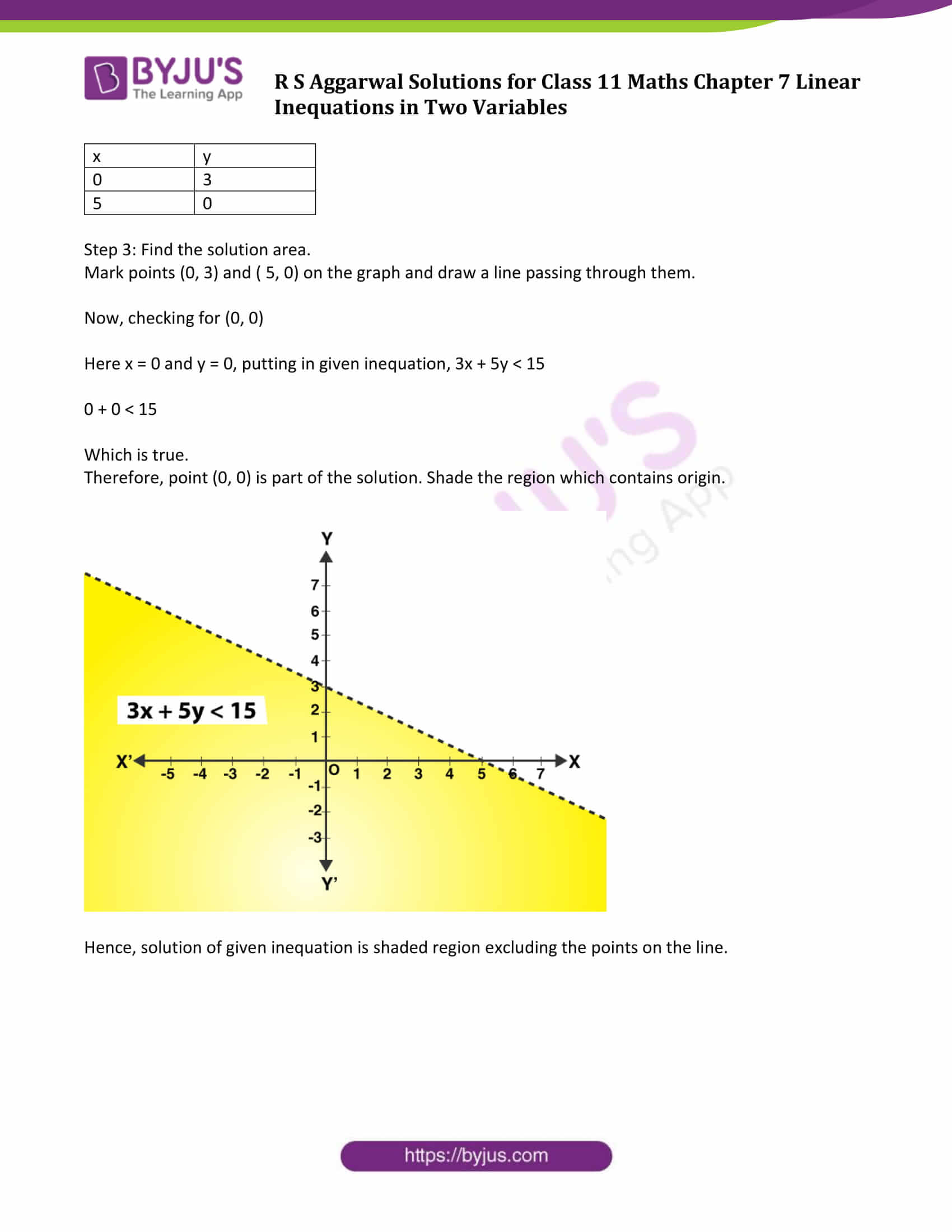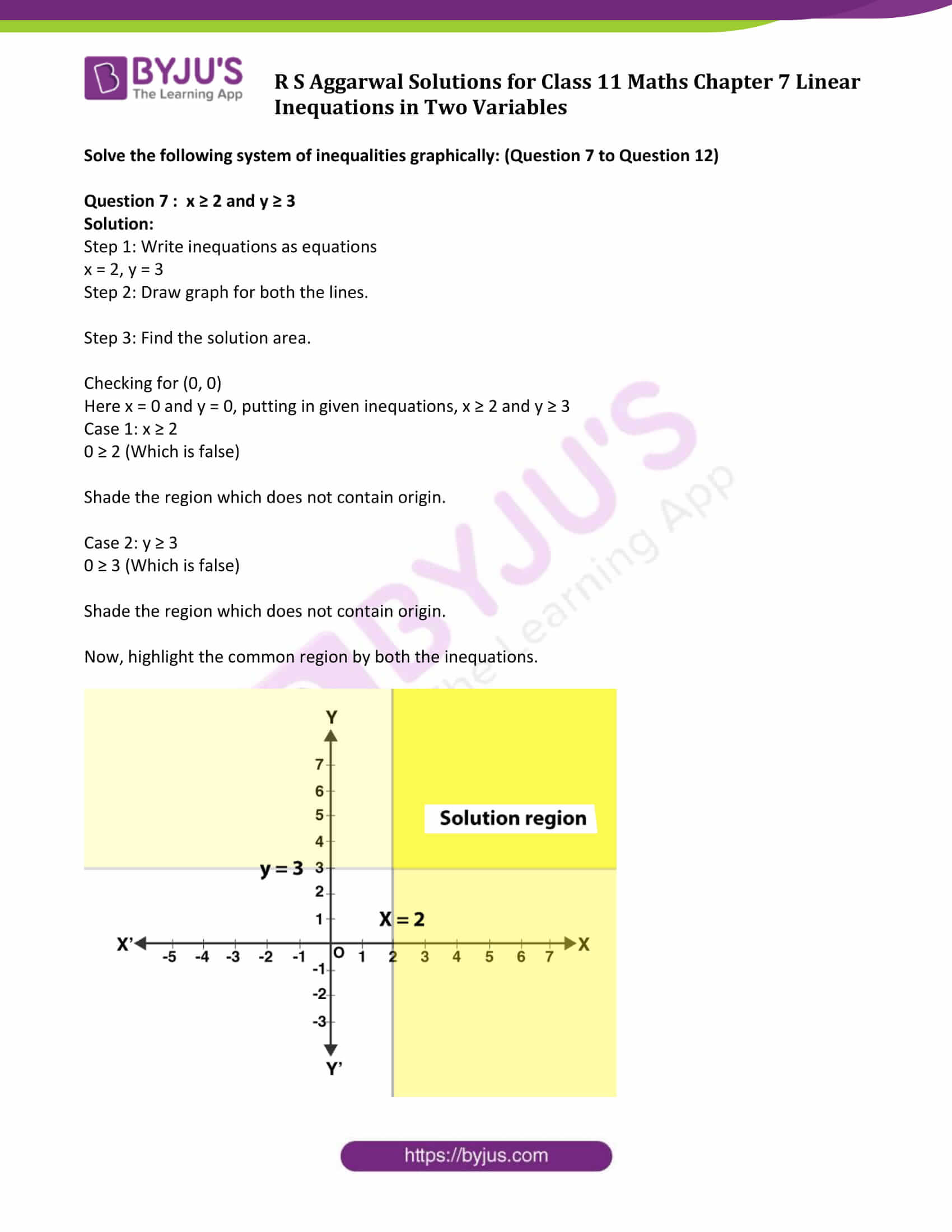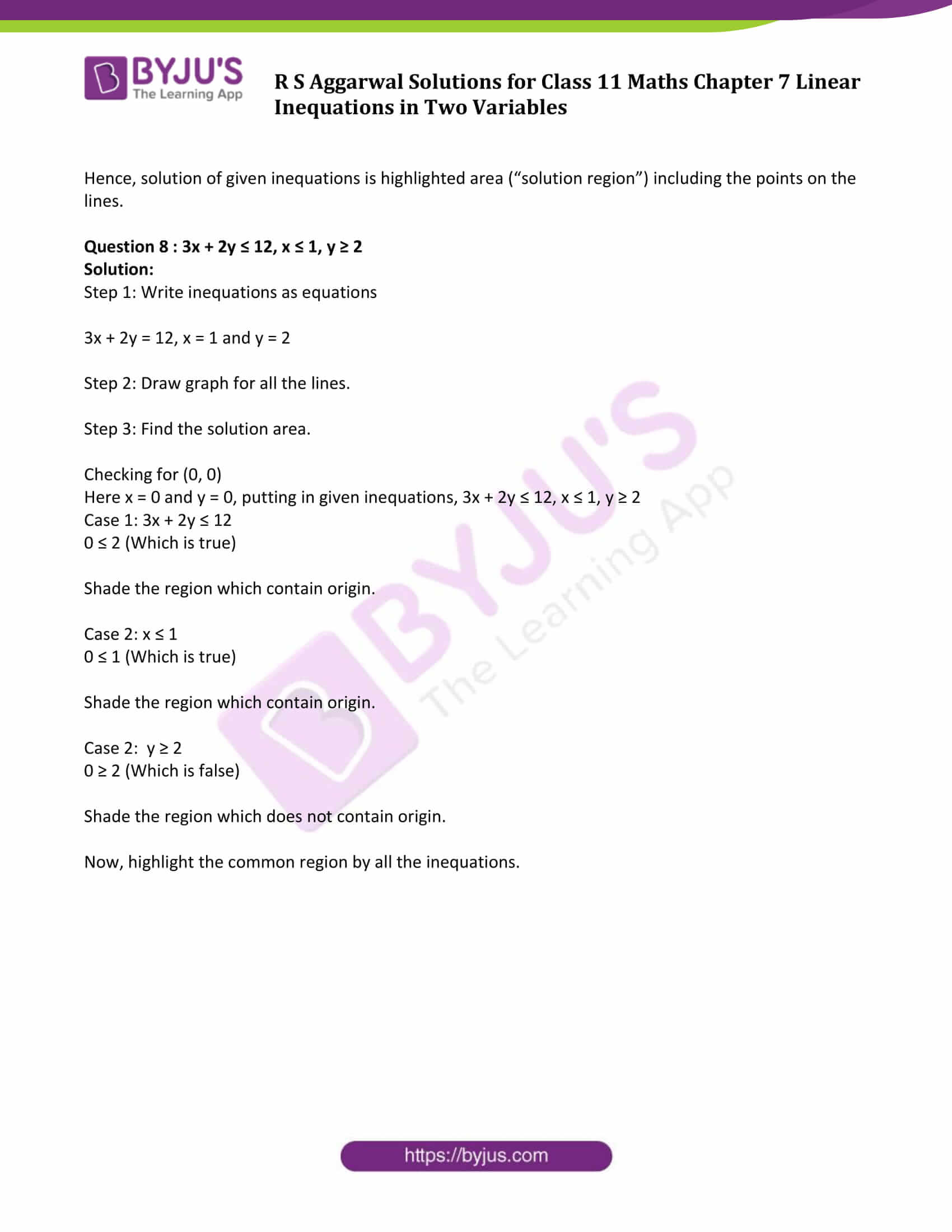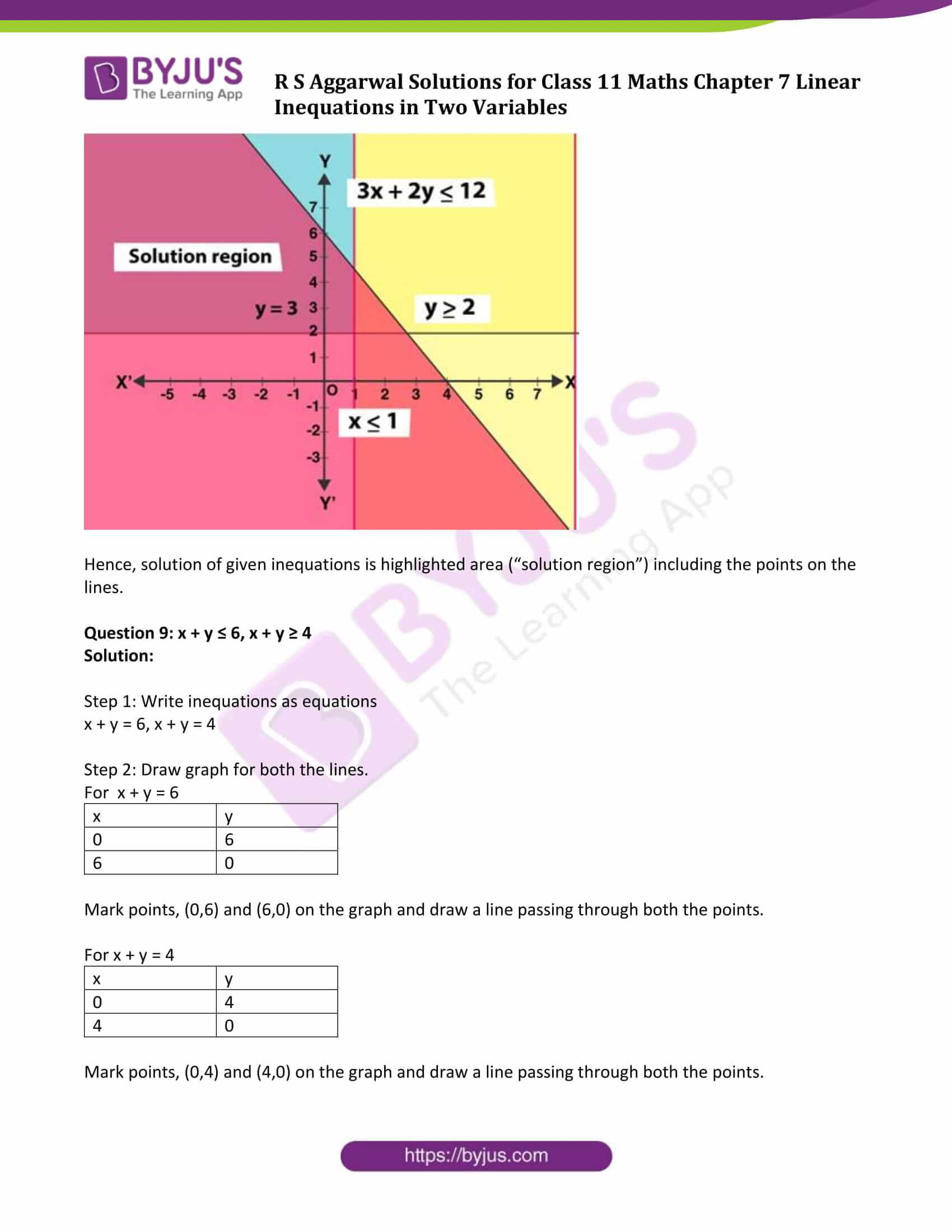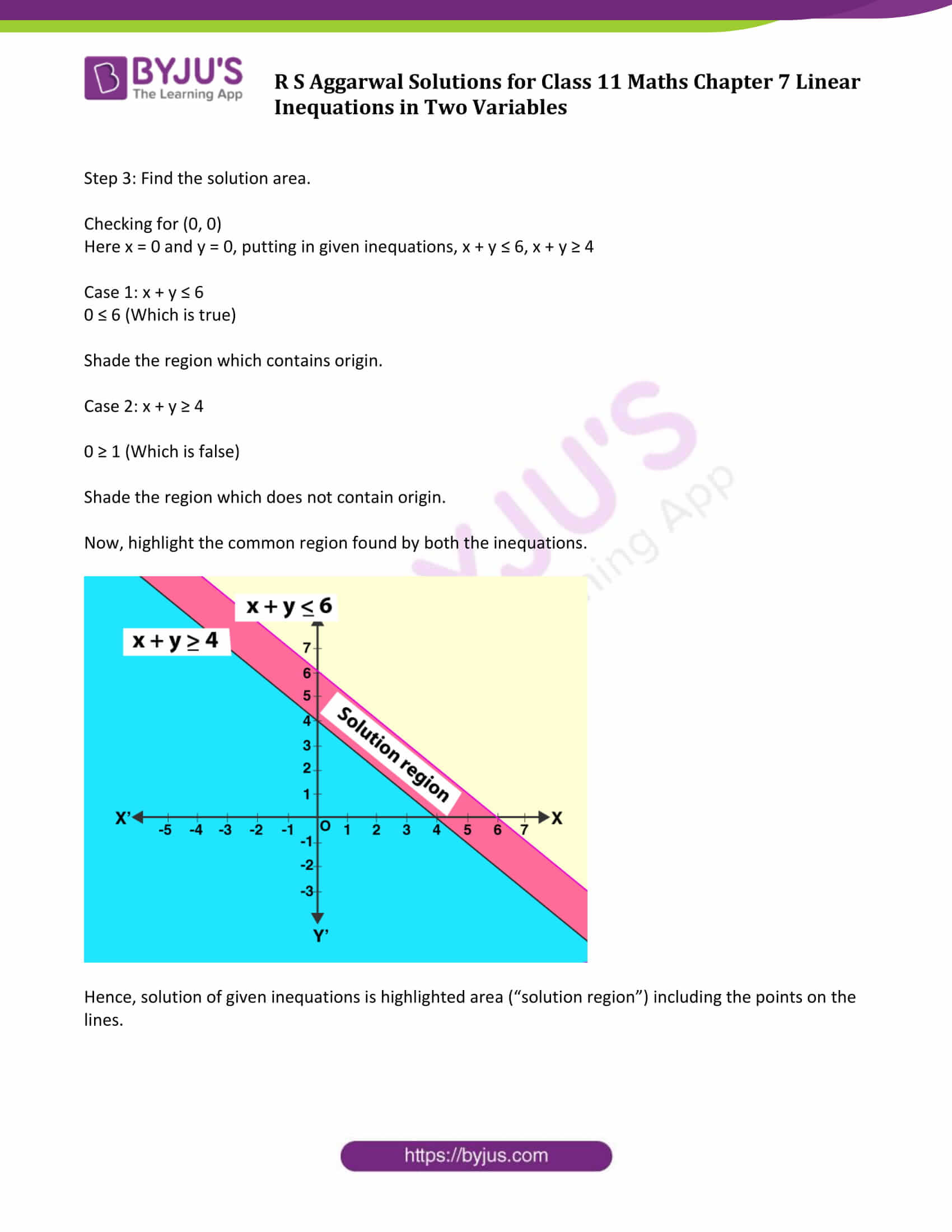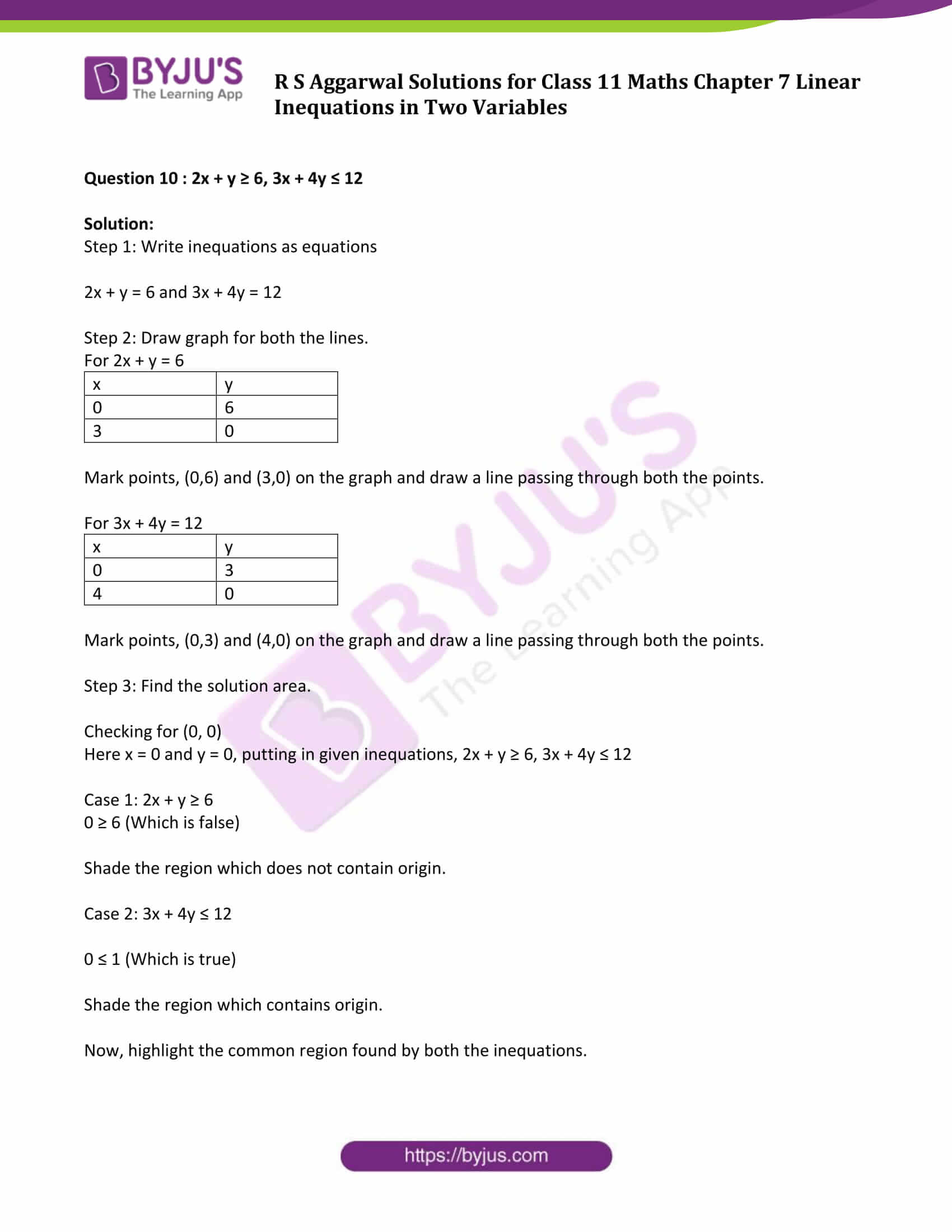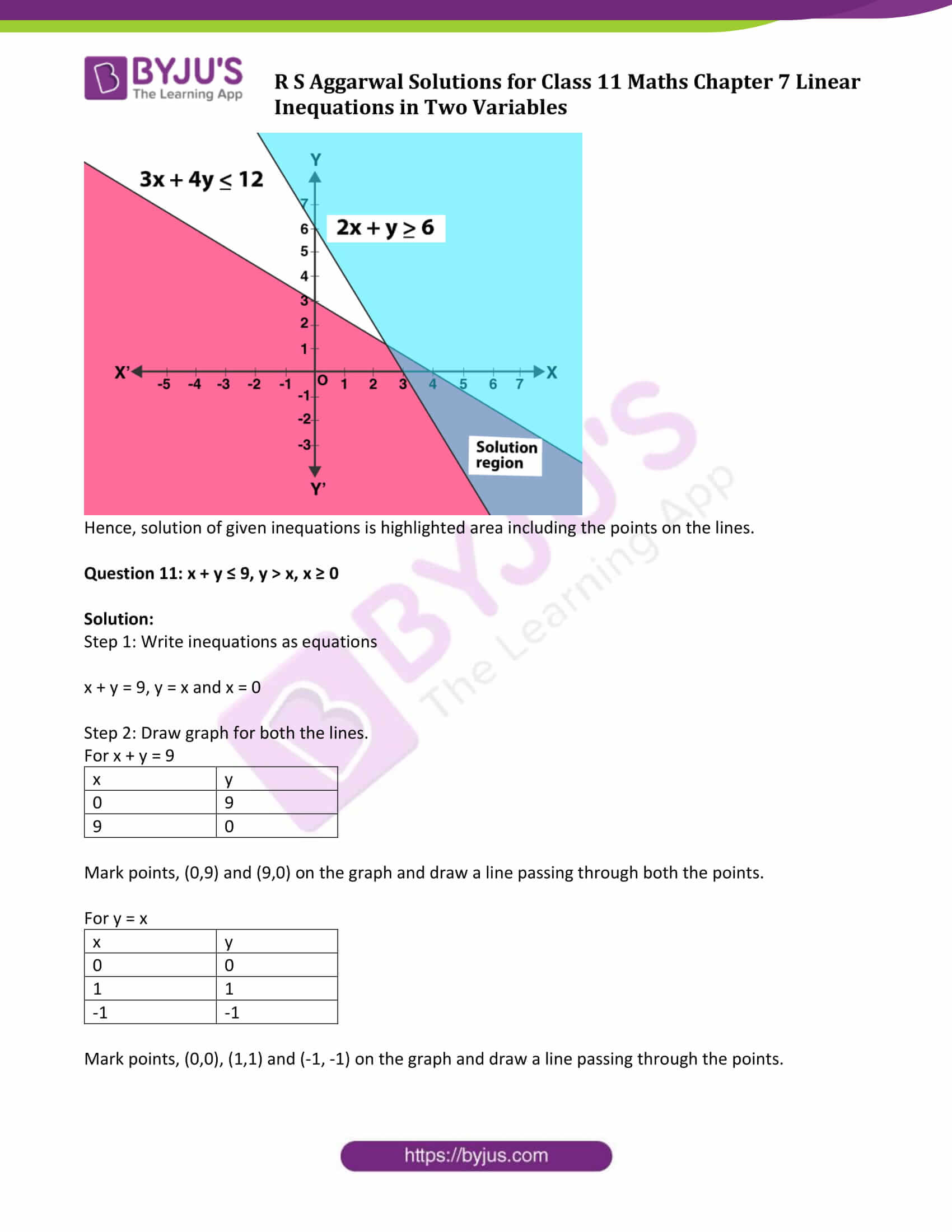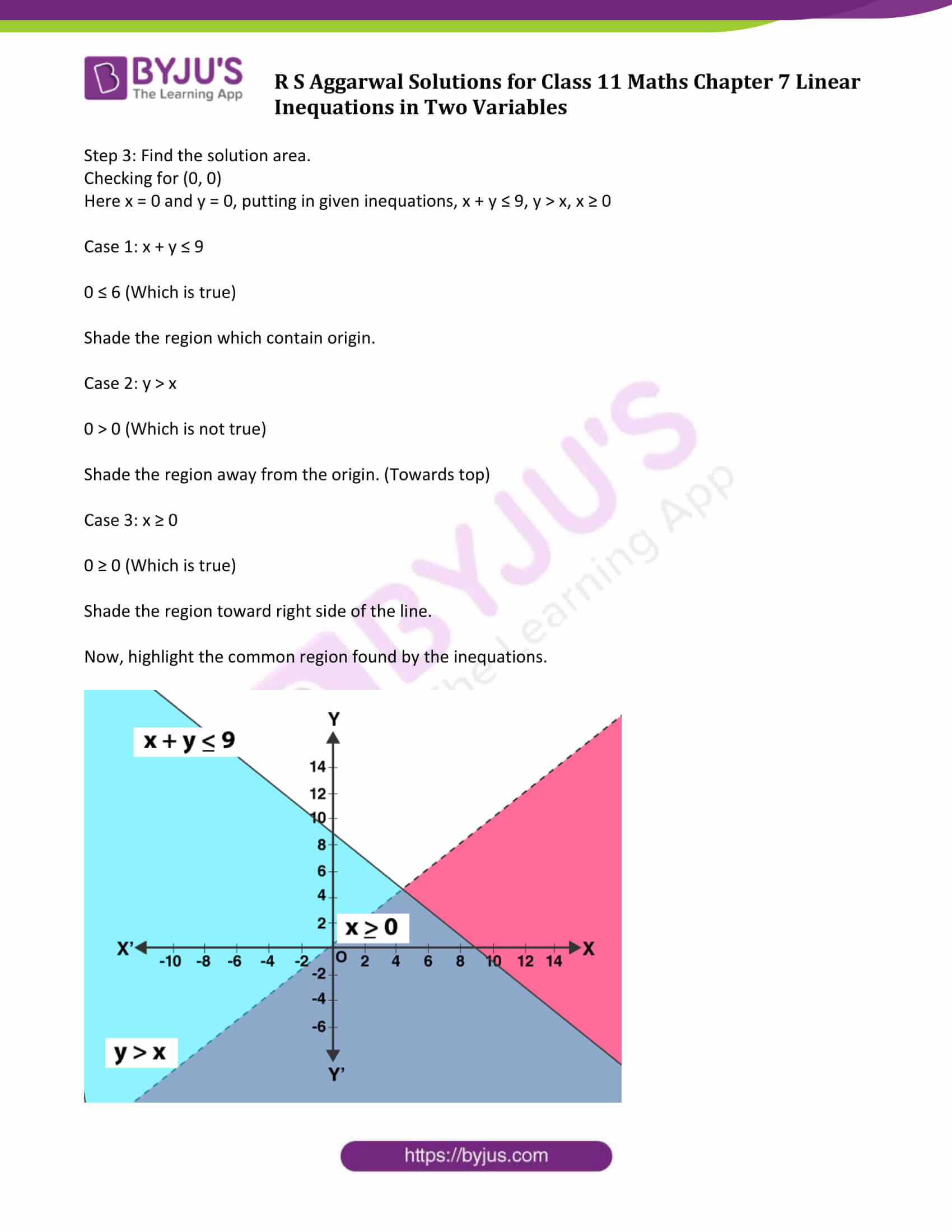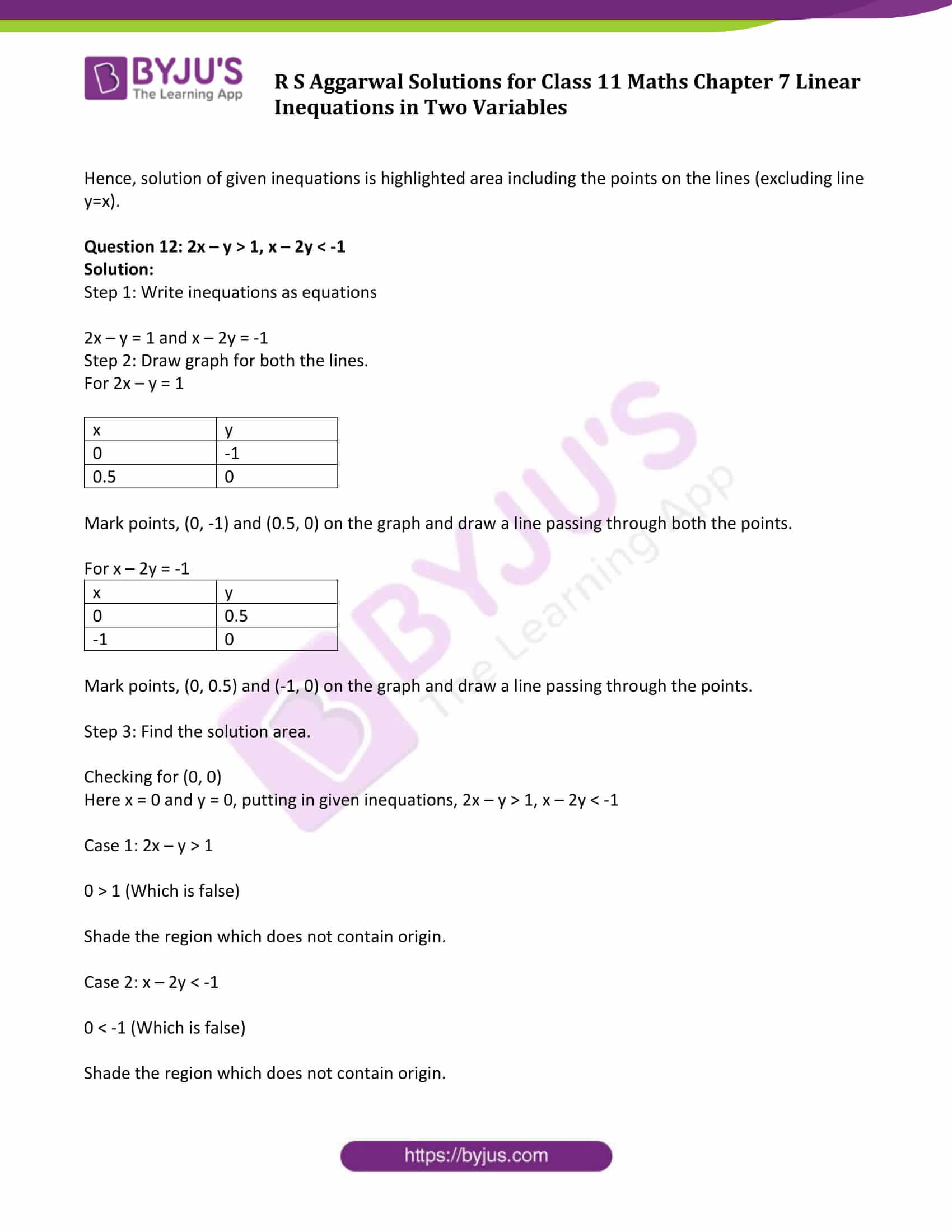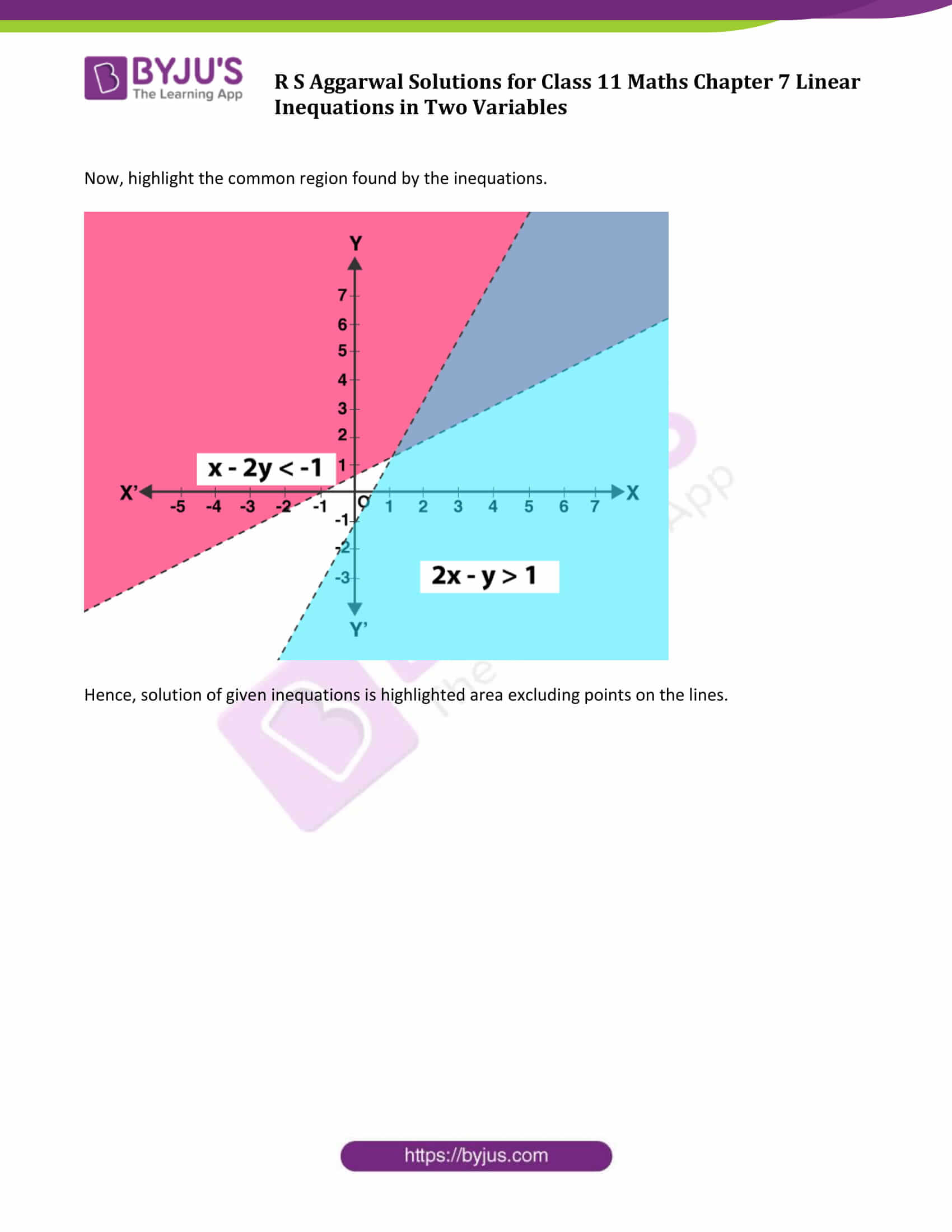### Access Answers to Maths R S Aggarwal Chapter 7 Linear Inequations in Two Variables

Exercise 7 Page No: 261

Draw the graph of the solution set of each of the following inequations: (Question 1 to Question 6)

Question 1: x + y ≥ 4

Solution:

Step 1: Convert into equation

x + y = 4

Step 2: Convert the equation in the slope-intercept form and find the solutions.

y = 4 – x …….(1)

Putting x = 0 in (1)

y = 4

Putting y = 0 in (1)

x = 4

 x y 0 4 4 0

Step 3: Find the solution area.

Mark points (0, 4) and (4, 0) on the graph and draw a line passing through them.

Now, checking for (0, 0)

Here x = 0 and y = 0, putting in given inequation

0 + 0 ≥ 4

0 ≥ 4

Which is false.

Therefore, point (0, 0) is not part of solution. Shade the region which does not contain origin.Hence shaded region including the points on the line is the solution of given inequation.

Question 2: x – y ≤ 3

Solution:

Step 1: Convert into equation

x – y = 3

Step 2: Write the equation in the slope-intercept form and find the solutions.

y = x – 3 …(1)

Putting x = 0 in (1)

y = -3

Putting y = 0 in (1)

x = 3

 x y 0 -3 3 0

Step 3: Find the solution area.

Mark points (0, -3) and (3, 0) on the graph and draw a line passing through them.

Now, checking for (0, 0) in given inequation x – y ≤ 3

Here x = 0 and y = 0

0 – 0 ≤ 3

0 ≤ 3

Which is true.

Therefore, point (0, 0) is part of the solution. Shade the region which contains origin.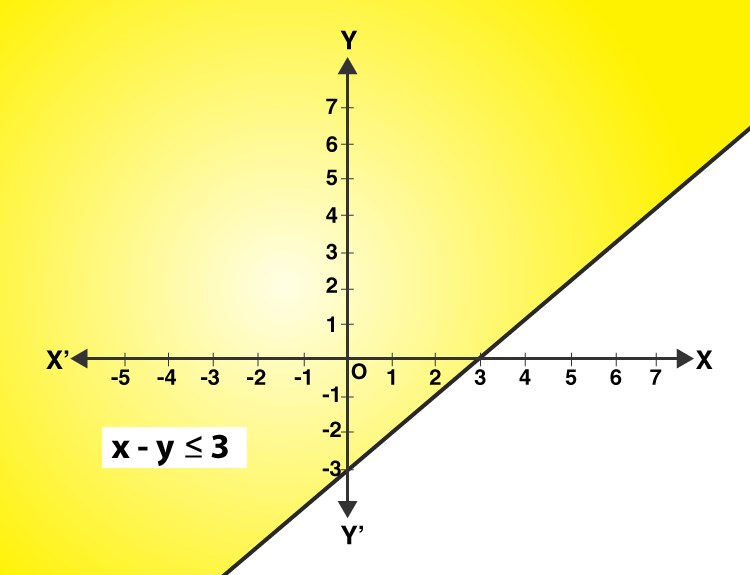Hence, solution of given inequation is shaded region including the points on the line.

Question 3: y – 2 ≤ 3x

Solution:

Step 1: Convert into equation

y – 2 = 3x

Step 2: Write the equation in the slope-intercept form and find the solutions.

y = 3x + 2 …(1)

Putting x = 0 in (1)

y = 2

Putting y = 0 in (1)

x = -2/3

 x y 0 2 -2/3 0

Step 3: Find the solution area.

Mark points (0, 2) and (-2/3 , 0) on the graph and draw a line passing through them.

Now, checking for (0, 0)

Here x = 0 and y = 0, putting in given inequation, y – 2 ≤ 3x

0 – 2 ≤ 0

-2 ≤ 0

Which is true.

Therefore, point (0, 0) is part of the solution. Shade the region which contains origin.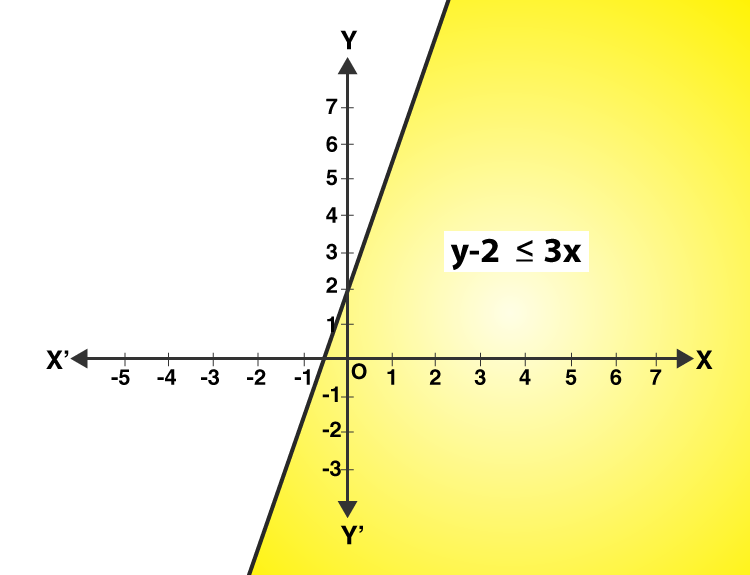Hence, solution of given inequation is shaded region including the points on the line.

Question 4: x ≥ y – 2

Solution: Step 1: Convert into equation

x = y – 2

Step 2: Write the equation in the slope-intercept form and find the solutions.

y = x + 2 ……(1)

Putting x = 0 in (1)

y = 2

Putting y = 0 in (1)

x = -2

 x y 0 2 -2 0

Step 3: Find the solution area.

Mark points (0,2 ) and ( -2, 0) on the graph and draw a line passing through them.

Now, checking for (0, 0)

Here x = 0 and y = 0, putting in given inequation, x ≥ y – 2

0 ≥ 0 – 2

0 ≥ -2

Which is true.

Therefore, point (0, 0) is part of the solution. Shade the region which contains origin.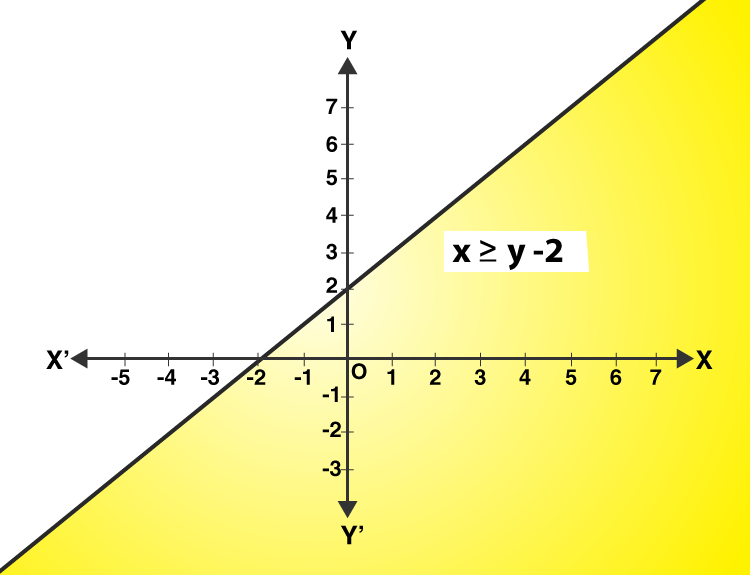Hence, solution of given inequation is shaded region including the points on the line.

Question 5: 3x + 2y > 6

Solution: Step 1: Convert into equation

3x + 2y = 6

Step 2: Write the equation in the slope-intercept form and find the solutions.

y = 1/2 (6 – 3x) …….(1)

Putting x = 0 in (1)

y = 3

Putting y = 0 in (1)

x = 2

 x y 0 3 2 0

Step 3: Find the solution area.

Mark points (0, 3) and ( 2, 0) on the graph and draw a line passing through them.

Now, checking for (0, 0)

Here x = 0 and y = 0, putting in given inequation, 3x + 2y > 6

0 + 0 > 6

Which is false.

Therefore, point (0, 0) is not part of solution. Shade the region which does not contain origin.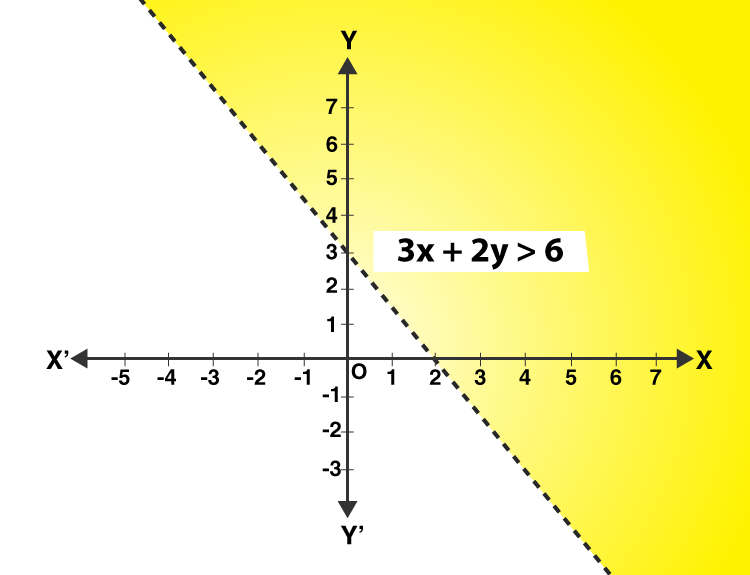Hence, solution of given inequation is shaded region excluding the points on the line.

Question 6: 3x + 5y < 15

Solution:

Step 1: Convert into equation

3x + 5y = 15

Step 2: Write the equation in the slope-intercept form and find the solutions.

y = 1/5 (15 – 3x) …(1)

Putting x = 0 in (1)

y = 3

Putting y = 0 in (1)

x = 5

 x y 0 3 5 0

Step 3: Find the solution area.

Mark points (0, 3) and ( 5, 0) on the graph and draw a line passing through them.

Now, checking for (0, 0)

Here x = 0 and y = 0, putting in given inequation, 3x + 5y < 15

0 + 0 < 15

Which is true.

Therefore, point (0, 0) is part of the solution. Shade the region which contains origin.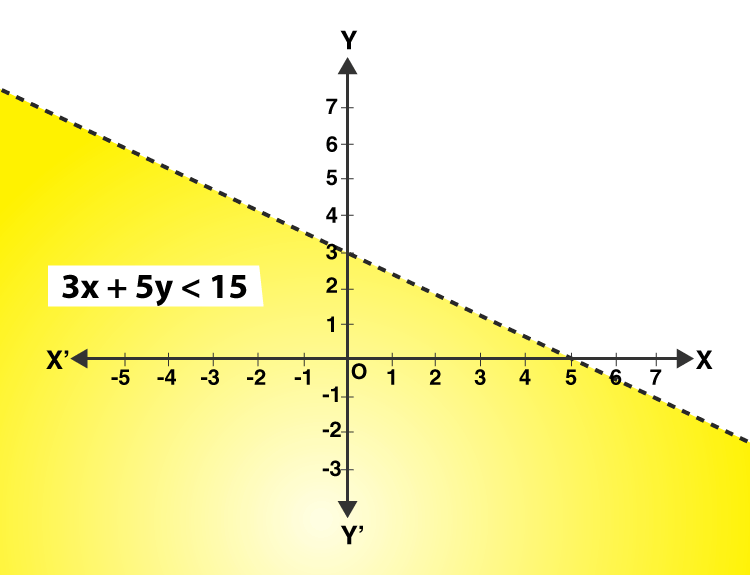Hence, solution of given inequation is shaded region excluding the points on the line.

Solve the following system of inequalities graphically: (Question 7 to Question 12)

Question 7 : x ≥ 2 and y ≥ 3

Solution:

Step 1: Write inequations as equations

x = 2, y = 3

Step 2: Draw graph for both the lines.

Step 3: Find the solution area.

Checking for (0, 0)

Here x = 0 and y = 0, putting in given inequations, x ≥ 2 and y ≥ 3

Case 1: x ≥ 2

0 ≥ 2 (Which is false)

Shade the region which does not contain origin.

Case 2: y ≥ 3

0 ≥ 3 (Which is false)

Shade the region which does not contain origin.

Now, highlight the common region by both the inequations.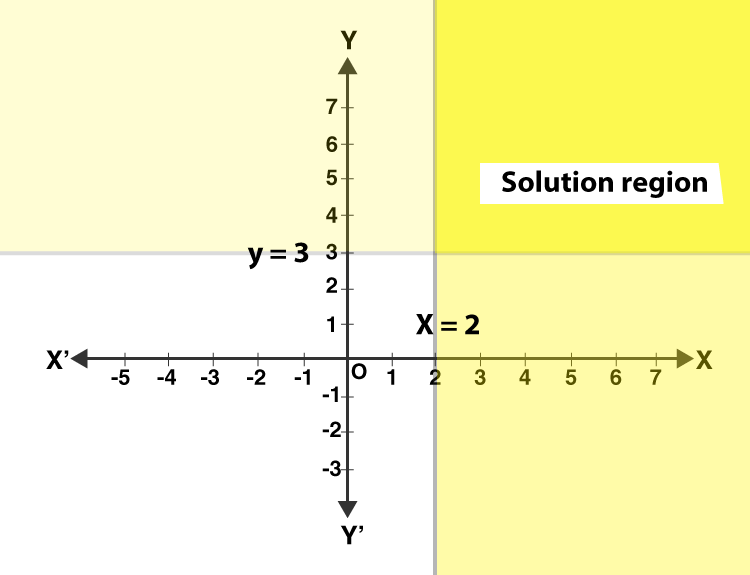Hence, solution of given inequations is highlighted area (“solution region”) including the points on the lines.

Question 8 : 3x + 2y ≤ 12, x ≤ 1, y ≥ 2

Solution:

Step 1: Write inequations as equations

3x + 2y = 12, x = 1 and y = 2

Step 2: Draw graph for all the lines.

Step 3: Find the solution area.

Checking for (0, 0)

Here x = 0 and y = 0, putting in given inequations, 3x + 2y ≤ 12, x ≤ 1, y ≥ 2

Case 1: 3x + 2y ≤ 12

0 ≤ 2 (Which is true)

Shade the region which contain origin.

Case 2: x ≤ 1

0 ≤ 1 (Which is true)

Shade the region which contain origin.

Case 2: y ≥ 2

0 ≥ 2 (Which is false)

Shade the region which does not contain origin.

Now, highlight the common region by all the inequations.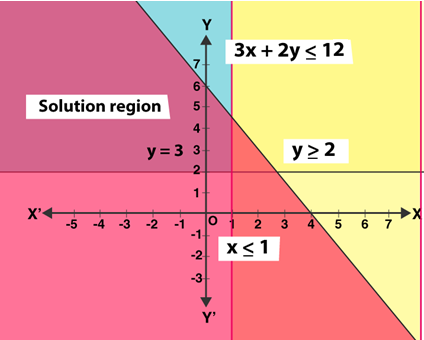Hence, solution of given inequations is highlighted area (“solution region”) including the points on the lines.

Question 9: x + y ≤ 6, x + y ≥ 4

Solution:

Step 1: Write inequations as equations

x + y = 6, x + y = 4

Step 2: Draw graph for both the lines.

For x + y = 6

 x y 0 6 6 0

Mark points, (0,6) and (6,0) on the graph and draw a line passing through both the points.

For x + y = 4

 x y 0 4 4 0

Mark points, (0,4) and (4,0) on the graph and draw a line passing through both the points.

Step 3: Find the solution area.

Checking for (0, 0)

Here x = 0 and y = 0, putting in given inequations, x + y ≤ 6, x + y ≥ 4

Case 1: x + y ≤ 6

0 ≤ 6 (Which is true)

Shade the region which contains origin.

Case 2: x + y ≥ 4

0 ≥ 1 (Which is false)

Shade the region which does not contain origin.

Now, highlight the common region found by both the inequations.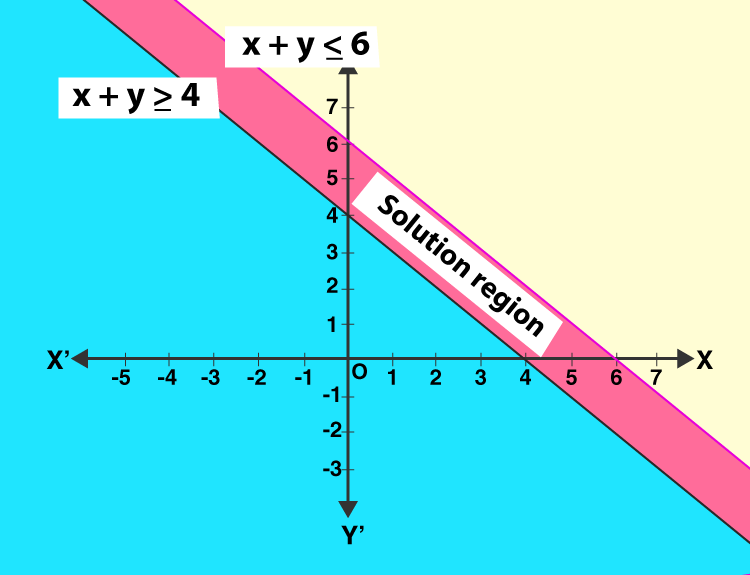Hence, solution of given inequations is highlighted area (“solution region”) including the points on the lines.

Question 10 : 2x + y ≥ 6, 3x + 4y ≤ 12

Solution:

Step 1: Write inequations as equations

2x + y = 6 and 3x + 4y = 12

Step 2: Draw graph for both the lines.

For 2x + y = 6

 x y 0 6 3 0

Mark points, (0,6) and (3,0) on the graph and draw a line passing through both the points.

For 3x + 4y = 12

 x y 0 3 4 0

Mark points, (0,3) and (4,0) on the graph and draw a line passing through both the points.

Step 3: Find the solution area.

Checking for (0, 0)

Here x = 0 and y = 0, putting in given inequations, 2x + y ≥ 6, 3x + 4y ≤ 12

Case 1: 2x + y ≥ 6

0 ≥ 6 (Which is false)

Shade the region which does not contain origin.

Case 2: 3x + 4y ≤ 12

0 ≤ 1 (Which is true)

Shade the region which contains origin.

Now, highlight the common region found by both the inequations.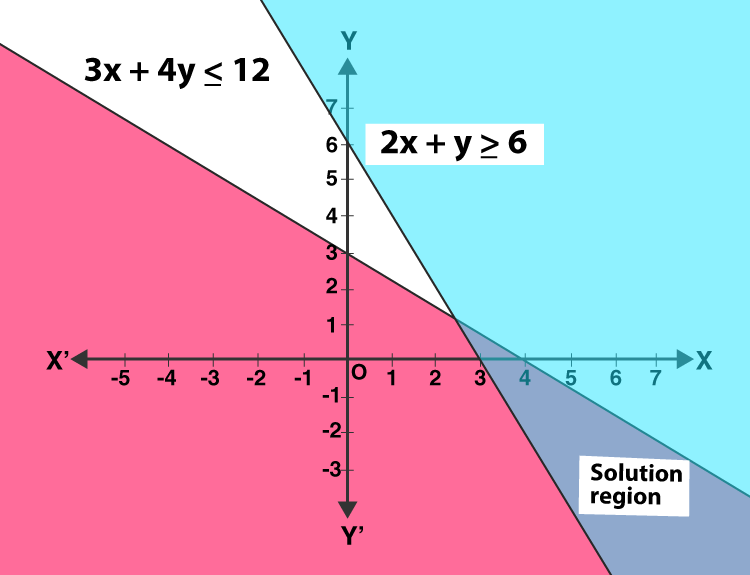Hence, solution of given inequations is highlighted area including the points on the lines.

Question 11: x + y ≤ 9, y > x, x ≥ 0

Solution:

Step 1: Write inequations as equations

x + y = 9, y = x and x = 0

Step 2: Draw graph for both the lines.

For x + y = 9

 x y 0 9 9 0

Mark points, (0,9) and (9,0) on the graph and draw a line passing through both the points.

For y = x

 x y 0 0 1 1 -1 -1

Mark points, (0,0), (1,1) and (-1, -1) on the graph and draw a line passing through the points.

Step 3: Find the solution area.

Checking for (0, 0)

Here x = 0 and y = 0, putting in given inequations, x + y ≤ 9, y > x, x ≥ 0

Case 1: x + y ≤ 9

0 ≤ 6 (Which is true)

Shade the region which contain origin.

Case 2: y > x

0 > 0 (Which is not true)

Shade the region away from the origin. (Towards top)

Case 3: x ≥ 0

0 ≥ 0 (Which is true)

Shade the region toward right side of the line.

Now, highlight the common region found by the inequations.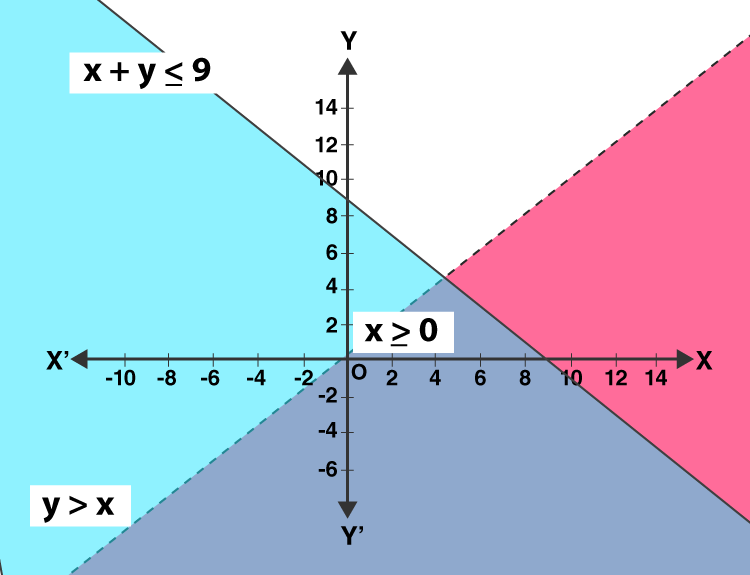Hence, solution of given inequations is highlighted area including the points on the lines (excluding line y=x).

Question 12: 2x – y > 1, x – 2y < -1

Solution:

Step 1: Write inequations as equations

2x – y = 1 and x – 2y = -1

Step 2: Draw graph for both the lines.

For 2x – y = 1

 x y 0 -1 0.5 0

Mark points, (0, -1) and (0.5, 0) on the graph and draw a line passing through both the points.

For x – 2y = -1

 x y 0 0.5 -1 0

Mark points, (0, 0.5) and (-1, 0) on the graph and draw a line passing through the points.

Step 3: Find the solution area.

Checking for (0, 0)

Here x = 0 and y = 0, putting in given inequations, 2x – y > 1, x – 2y < -1

Case 1: 2x – y > 1

0 > 1 (Which is false)

Shade the region which does not contain origin.

Case 2: x – 2y < -1

0 < -1 (Which is false)

Shade the region which does not contain origin.

Now, highlight the common region found by the inequations.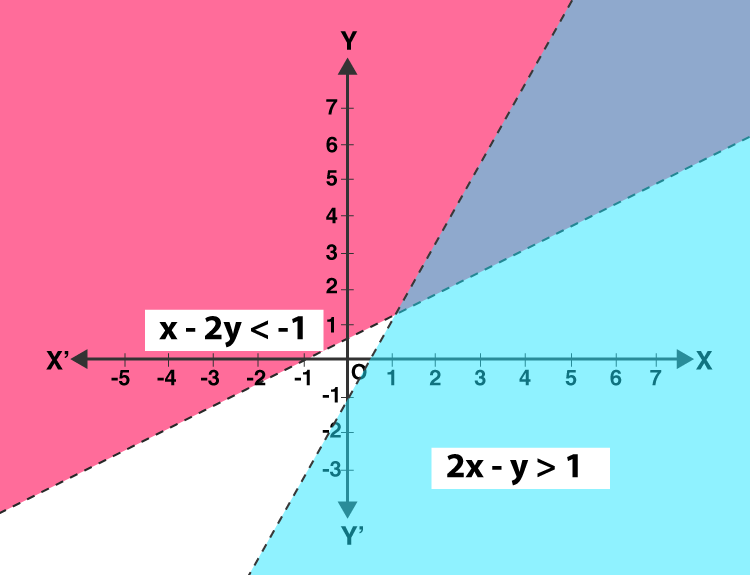Hence, solution of given inequations is highlighted area excluding points on the lines.

## R S Aggarwal Solutions for Chapter 7 Linear Inequations in Two Variables

R S Aggarwal solutions given here contain answers to all the questions presented in this chapter. Here, students will learn how to find the solution for linear inequations in two variables using graphical method. At the end of this chapter, students will be able to exhibit graphically the solution set of any linear inequation at their own pace.